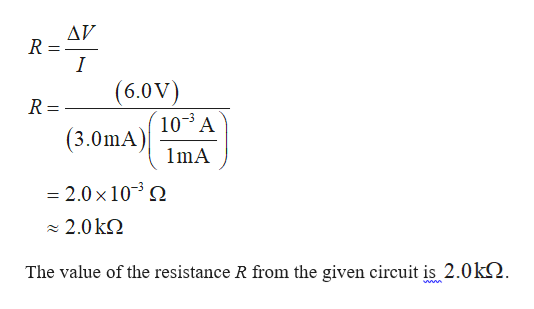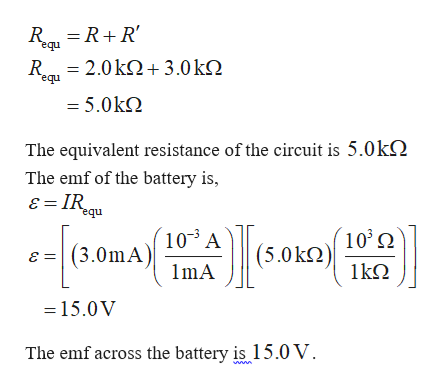Question
7 views

For the circuit shown in Figure P18.48, the voltmeter
reads 6.0 V and the ammeter reads 3.0 mA. Find (a) the value
of R, (b) the emf of the battery, and (c) the voltage across the
3.0 - kΩ resistor. (d) What assumptions did you have to make to
solve this problem?

check_circle

Step 1

Formula to calculate the value of the resistance Rhelp_outlineImage TranscriptioncloseAV (6.0V) 10 A (3.0mA) R = %3D 1mA = 2.0 x 10- O - 2.0 kQ The value of the resistance R from the given circuit is 2.0k. fullscreen
Step 2

b).

Formula to calculate the equivalent ...help_outlineImage TranscriptioncloseR = R+R' equ R = 2.0 k2+ 3.0kQ equ = 5.0kQ The equivalent resistance of the circuit is 5.0kN The emf of the battery is, ɛ = IRqu nba 10³ A ´10°2 (3.0mA) (5.0kN ɛ = 1kΩ 1mA = 15.0V The emf across the battery is 15.0 V. fullscreen

### Want to see the full answer?

See Solution

#### Want to see this answer and more?

Solutions are written by subject experts who are available 24/7. Questions are typically answered within 1 hour.*

See Solution
*Response times may vary by subject and question.
Tagged in

### Physics